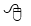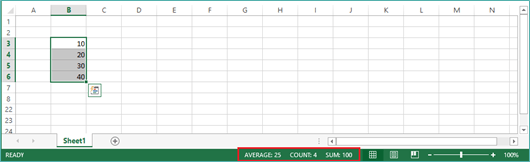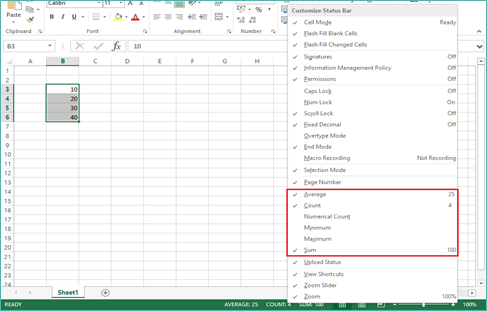There are so many formulas in Excel and some of these formulas can take quite a while to build. However, if you need a quick calculation on your data you can choose one of the six taskbar calculations where you don’t even need to enter the formula.

Do I need to enter a formula to calculate cells in Excel?

No, not at all. There may be occasions when you need to check the current total for a group of cells but you may not be ready to create the formula at this stage.

How do I calculate a total without entering a formula?

When a number of values have been entered in a worksheet and these values have been selected a total will be displayed in the taskbar at the bottom of the screen. More often than not the total displayed will be a sum of the cells but this can be changed by right clicking the sum calculation and selecting another calculation option from the list e.g. Average or Count. The method explained below relates to Excel 2013 but the same principal works in all versions of Excel.Select the figures in the worksheet that you want to use in the calculation.

The taskbar at the bottom of the worksheet will now display the 3 most popular calculations – Average, Count and Sum.You can also access another 3 calculations by right clicking the taskbar and selecting one of the options from the shortcut menu. If you click one of the additional calculations, this will also appear on the taskbar and will be available on the taskbar in each new worksheet or workbook that you open.